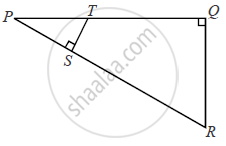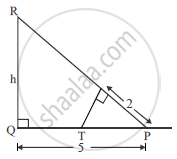Advertisement Remove all ads

# In the given figure, ∠PQR = ∠PST = 90° ,PQ = 5cm and PS = 2cm. (i) Prove that ΔPQR ~ ΔPST. (ii) Find Area of ΔPQR : Area of quadrilateral SRQT. - Mathematics

Sum

In the given figure,  ∠PQR = ∠PST = 90°  ,PQ = 5cm and PS =  2cm.
(i) Prove that ΔPQR ~ ΔPST.
(ii) Find Area of ΔPQR : Area of quadrilateral SRQT.Advertisement Remove all ads

#### Solution

To prove ΔPQR ∼ ΔPST

In ΔPQR and ΔPST

∠PQR = ∠PST = 90°

∠P = ∠P               ( common )

∴ ΔPQR ∼ ΔPST               {by AA axiom}(ii) ("Area of PQR ")/("Area of Quadrilateral") =

("ar" (ΔPQR))/("ar" (ΔPST )) = (1/2 xx PQ xx QR )/(1/2 xxPS xx ST) = 5/2 xx 5/2

("ar" (ΔPQR))/("ar" (ΔPST )) = 25/4 [ ∵ (PQ)/(PS) = (QR)/(ST) ]

Taking the reciprocals on both sides

("ar" (ΔPST ))/("ar" (ΔPQR)) = 4/25

Now deducting both sides by 1

 therefore 1 - ("ar" (ΔPST ))/("ar" (ΔPQR)) = 1-4/25

("ar" (ΔPQR )- "ar" (ΔPST)) /("ar"(ΔPQR)) = 21/25

⇒ ("Area of quadrilateral")/("ar"(Delta PQR)) = 21/25

Is there an error in this question or solution?
Advertisement Remove all ads

#### APPEARS IN

Advertisement Remove all ads

#### Video TutorialsVIEW ALL 

Advertisement Remove all ads
Share
Notifications

View all notifications

Forgot password?
Course